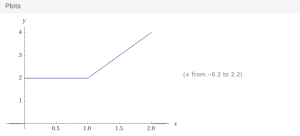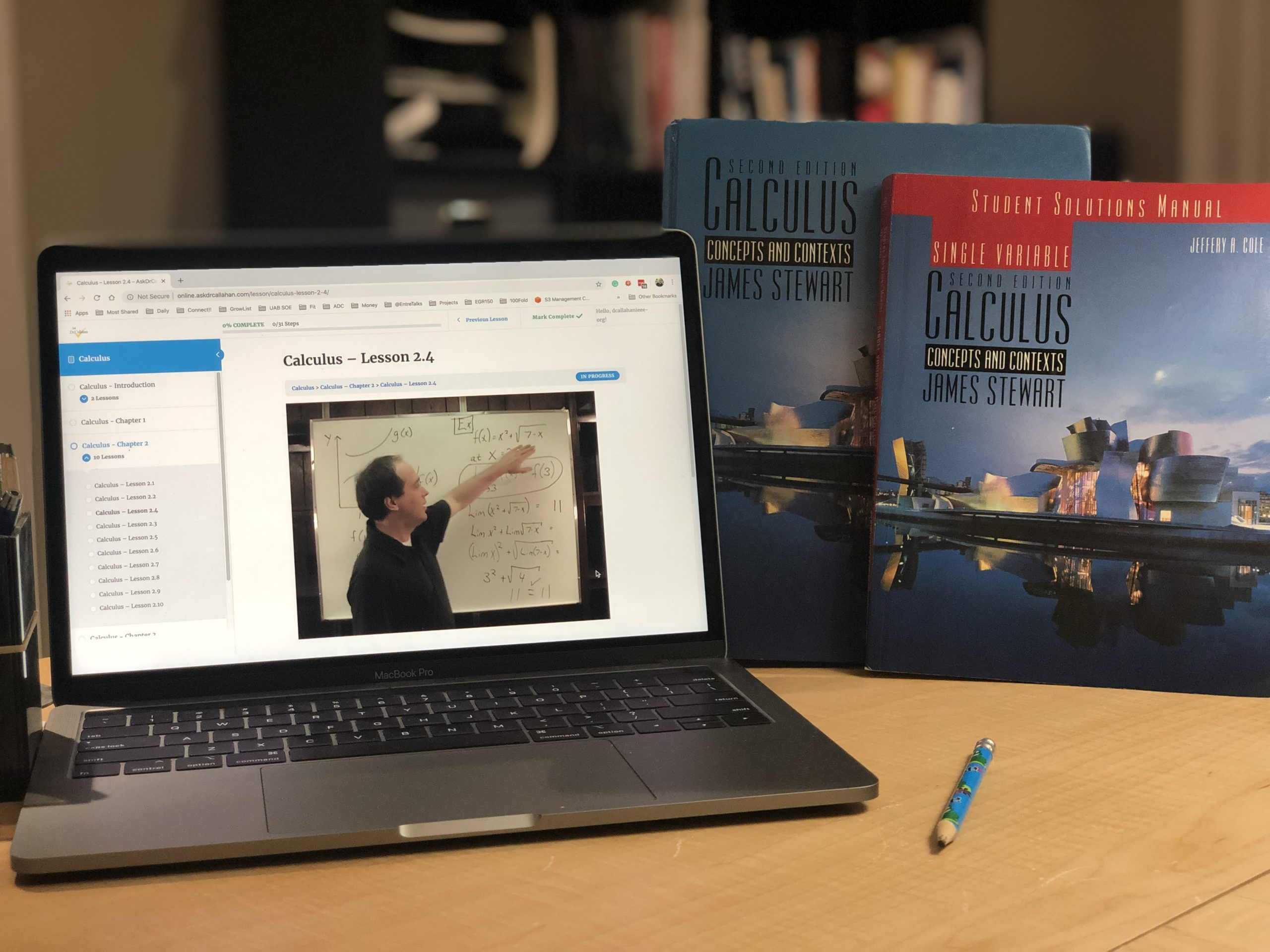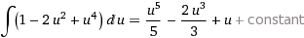Posted on

## Calculus Ch1.1 #44, 46, 48, 50

Here are the solutions to these even-numbered problems.

Calc1-Ch1.1#44-46-48-50.pdf (The PDF has #49 instead of #50. See below for #50)

Chapter 1.1 #50

What you are looking for is a function like
C = f(x) where C is cost and x is mile
If 0 <= x <= 1, C=2
If 1 < x < =2, C = 2 + 0.2(10(x-1))
Using Wolfram here is what it looks likePosted on

## Calculus 1 Course Description for TranscriptIf you are looking for details for a transcript for Calculus 1,  this should do the trick.

Calculus is about change. The tools of calculus allow us to model change. Once we have equations for something, we can use calculus to see the impact of change on the system.

In Algebra, we learned how to generalized basic arithmetic to show relationships. So, instead of saying 2 + 2 = 4, we might say 2 + x = y, allowing a more general equation that shows a relationship between x and y.

In Geometry (which also includes trigonometry), we studied the relationships of shape.

In Calculus, we study continuous change. For example, our car gets so much gas milage which is impacted by the car’s weight. But the longer you drive, the less gas you have and therefore the less weight. So the weight of the car changes over time, therefore impacting the use of fuel over time.

Calculus is a necessary tool for any science or engineering. It is heavily used in physics and engineering and found in biology, business, social sciences, etc. No matter what we are studying, the only constant thing is change.

Calculus is broken up into two areas – Derivative and Integral Calculus. This course covers derivative calculus, which is equivalent to most Calculus 1 courses at most universities. Calculus 2 covers integral calculus.

Students who complete Calculus are prepared to enter college and should be able to step into a college-level Calculus 1 or Calculus 2 course – depending on how the individual university defines its course. Calculus is not required for the ACT and the SAT.

Topics Covered in this Course:

• Limits and limit laws
• Continuity
• Derivatives
• Differentiation rules
• Application of differentiation
• Antiderivatives
Posted on

## Calculus Textbook Evaluations

Look for the following material or heading in the contents.

• Limits and Derivatives
· Limit of a function
· Calculating limits
· Limit laws
· Continuity
• Differentiation Rules
· Rates of change
· Derivative rules (polynomials and exponentials)
· Product rule
· Quotient rule
· Derivatives of trigonometric functions
· The chain rule
· Implicit differentiation
· Derivatives of logarithmic functions
• Applications of Differentiation (subtitles may differ widely here)

Notes for use: This course should be equivalent to a Calculus One course at a local university. If you know what school your child plans to attend, find out which textbook they use and go with it. In fact, ask for a syllabus and sample tests. A good deal of information can be gleaned from the UAB website on the screening test and the UAB test bank. (See the resources at the end of the chapter.)

Also, note that these books are often written for Calculus I, II, and III. So your text will be a lot bigger than what you will teach in high school. Also, if you do go with a college textbook you will pay a hefty price. An alternative is to find the previous edition on eBay – much cheaper with probably little change in material.

Posted on

## Calc1 Test 4 / Final Exam #6c

Looks like you have a simplification error in the second derivative. You have:

f”(x)=12x^2-4=4(x-1)
But it should be
f”(x)=12x^2-4=4(3x^2-1)
then the intervals for concavity all change.
Am I missing something? Thank you so much for your good product and wonderful support!
You are correct. Thanks for pointing that out.
See the plot of the original equation (a good idea is to look at plots when things are not working out)
From here we can see how it is shaped.
Now if we look at the second derivative, it should beand look like
which has rootsand looks like
The concavity chart will now show up, down, and up but around the pointsPosted on

## Calculus Test 4 Sections 4.9-5.8 – #3, #8b, #9a, #9b, #10b questions

Question from Beverly:

#3 –  on the test copy I have says to use three equal subintervals.  But in the handwritten solution, it appears that they used 6. If the student uses 3 subintervals wouldn’t the ∆X be 2 and the right endpoint sum be 0.2, left end point be 39.8, and midpoint be 15.8?

Answer from Dr Callahan – That is correct – in the solution we used an interval of 1 and it should have been 2.

#8b – The handwritten solution has dV= √t and V = t^1.5.  Shouldn’t V = 2/3*t^1.5? With that, our final solution came out to be 3.39.

Answer from Dr Callahan – That is also correct – we have an error in our solution.

#9a – Instead of factoring the cos^5x into (cos^2x)(cos^2x)cosx, my student used the Form 74 from the Table of Integrals followed by Form 68. But he didn’t get the same answer as he would have solving it as we learned in 5.7 (p. 403) like the written solution did. Why don’t the two methods yield the same answer? I’m thinking it has to do with the limits of the integral, but it’s been 25 years since I had calculus, and I’m struggling with explaining the why on this one.

Answer from Dr Callahan – Yes you should get the same answer – but I noticed an error in our solution!!!! When you integrate we added a 4. Should be as below#9b – In the handwritten solution it looks like it evaluates 3*ln 3 as 9.89, but isn’t it 3.3? I believe the answer would be -0.17 if 3.3 is correct.

Answer from Dr Callahan –  Yes it should be 3.3

Extra Credit – The solution just substituted into the Form #95.  Did you not expect them to continue to solve the remaining integral or just stop with that first substitution?

Answer from Dr Callahan – Yes we just stopped there because that was the challenging part!

Posted on

## Calculus Ch2.2 Test 1 #7

Question from Carl:

The question on the test has one slight variation from the book:

f (x)  =   x     if     -1< x<1
the text book shows

f (x)  =   x     if     -1< x<1

Please clarify if the teacher syllabus is incorrect.  We’re finding it is not possible to be both (0,1) and (1,1)…thus only (0,1) is solid and (1,1) is open on the graph.

Good catch – you are correct – cannot be both. The question should say

f (x)  =   x     if     -1< x<1

Posted on

## Calculus Ch2.2 #4

The answers are not given in the book since this is even, so here they are:

a) 3

b) 4

c) 2

d) does not exist

e) 3

Posted on

Question from Alyanya:

Hello,
I am looking for a math course for my daughter who just finished her sophomore year of high school. She received a 97 in  Algebra 2 Pre-AP and was planning on taking  Pre-Calculus Pre-AP next year.  However we have decided to home school next year because of our travel schedule, so I’m looking for a course for her.  I noticed that you don’t have a separate Pre-Calculus class.  In your opinion would it be ok for her to go straight to the Calculus class?  She’s a very strong student, but I wouldn’t want her to take something that she is not prepared for.
Any input would be appreciated.

My guess is she would be OK as long as she has had some trig.
I would first have her go to out online textbookhttp://www.mhhe.com/math/precalc/barnettcat7/student_index.mhtml
and take the chapter quizzes of each chapter, 1-8.
This will show any weak spots. If she has any, a quick study of those sections should help her get ready.

Hope this helps.

Posted on

## Will Calculus Be Too Difficult For Me To Teach?

Question from Donna:

But I’m wondering if calculus will be too difficult for me to teach. Trig was a bit of a challenge for me, just wondering what your ideas were. Thanks